2D line representations

# 1. Introduction

A two-dimensional line is one of the most fundamental geometric objects. Depending on the subject area and concrete application, there are different possibilities how to represent a 2D line mathematically, each with its own pros and cons. This article presents the main different alternative representations. It describes the meaning of each representation and how to create the particular 2D line form from two given points. Further, it shows the geometric interpretation of each line form and how to convert one line representation form into another.

Following line representation equations are addressed:

• Slope-intercept form
• Point-slope form
• Two-point form
• General form
• Standard form
• Parametric form

In the following, it is assumed that the two points p0 and p1 lie on the two-dimensional line and have coordinates p0 = (x0, y0) and p1 = (x1, y1).

# 2. Slope-intercept form

The Slope-intercept form of a line is probably known from school math. It is defined by a slope (the steepness of the line) and the coordinate where it intersects the y coordinate axis.
The slope, denoted by m, is the rate between change in y to the change in x, thus m = Δy / Δx. The y-intercept, denoted as b, is the y coordinate of the intersection between line and y-coordinate axis. The equation of a line in slope-intercept form is:

y = m*x + b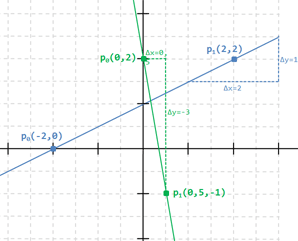Example:
The figure on the right shows two lines as an example.

The blue line has for each step into the x-direction, a half step into the y-direction, so its slope is m = 0.5. Also the intersection with the y-coordinate is at (0, 1), so the to y-intercept is b = 1. The equation of the blue line in slope-intercept form is y = 0.5 * x + 1.

For the green line to slope m is -6 (for each step to the right, there are 6 steps down) and b = 2, so the line equation is y = -6 * x + 2.

Given the two points p0 = (x0, y0) and p1 = (x1, y1), how to retrieve the slope-intercept form?
The slope m is the change in y divided by the change in x, so the coordinates of both points can be directly used to calculate m:
m = Δy / Δx = (y1 - y0) / (x1 - x0). Plugging it into the equation gives then:
y = (y1 - y0) / (x1 - x0) * x + b.
To find the y-intercept, the formula is changed so that b is isolated:
b = y - (y1 - y0) / (x1 - x0) * x. Now just plug in any of the two points for x and y to calculate b.

Example: Finding the equation of the blue line with points p0 = (-2, 0) and p1 = (2, 2).
m = (y1 - y0) / (x1 - x0) = (2 - 0) / (2 - (-2)) = 2 / 4 = 0.5.
b = y - m * x = y - 0.5x. Plugging in p1 = (2, 2) gives b = 2 - 0.5 * 2 = 1.
Summarized: y = 0.5 * x + 1

The main drawback of the slope-intercept form is that vertical lines cannot be represented (the slope m would be infinite). So this is a special case where the line equation x = a is used where a is the intersection of the line with the x-coordinate axis.
E.g x = 2 is the vertical line going through points (2, 0). This makes sense as every single point of this line has always an x-coordinate of 2.

# 3. Point-slope form

The point-slope form is related to the slope-intercept form. Instead of using slope m and y-intercept b to define the line, the slope m and any point on the line p0 is used.
The equation of a line in point-slope form is:

y - y0 = m * (x - x0)

It's just a more general from of the slope-intercept form because if p0 is chosen as y-intercept = (0, b), then we get y - b = m * (x - 0) => y = m*x + b.
The graphical interpretation of the point-slope form is also straight-forward as the slope m and one point of the line are directly visible in the equation.
Given two points p0 = (x0, y0) and p1 = (x1, y1) on the line, the retrieval of the point-slope form is as follows:
First calculate the slope m with m = (y1 - y0) / (x1 - x0). Then just plug in any of the two points.

Example: Point-slope form of line with points p0 = (-2, 0) and p1 = (2, 2).
m = (y1 - y0) / (x1 - x0) = (2 - 0) / (2 - (-2)) = 2 / 4 = 0.5.
y - y0 = 0.5 * ( x - x0).
Plugging in p1 = (2, 2) gives y - 2 = 0.5 * (x - 2). This can easily be changed to the slope-intercept form: y = 0.5 * x - 0.5 * 2 + 2 = 0.5 * x + 1.

# 4. Two-point form

Also the two-point form is closely related to slope-intercept and point-slope form - here two points p0 and p1 directly define the line. The equation of a line in two-point form is:

y - y0 = (x - x0) * (y1 - y0) / (x1 - x0)

The relation to the point-slope form is more then obvious as (y1 - y0) / (x1 - x0) is just slope m.

# 5. Standard form or Cartesian form

The standard form of a line has following equation:

a * x + b * y = c

This raises several questions: What is the geometrical interpretation of such equation? Is there a way to transform the equation to another type of line representation? And how to retrieve such an equation from two points? Let's start with the geometrical interpretation topic.

The geometrical interpretation is that vector a and b are the components of the unit vector which is perpendicular to the line and c is the (perpendicular) distance from the line to the origin.
However, this is only true if (a, b) is already a unit vector, thus if it the length of this vector is 1. Otherwise, the components has to be normalized by dividing all components of the equation be the length of (a, b) which is sqrt(a2 + b2).Example:
The figure on the right shows the line with equation 3 * x + 4 * y = 6.

Because the vector (3, 4) is not a unit vector, the equation must be divided by the vector length
which is sqrt(32 + 42) = sqrt(9 + 16) = sqrt(25) = 5.

This gives 3 / 5 * x + 4 / 5 * y = 6 / 5 which is equal to 0.6 * x + 0.8 * y = 1.2.

Note that the length of vector (a, b) is 1 because sqrt(0.62 + 0.82) = sqrt(0.36 + 0.64) = 1,
so the c = 1.2 on the left side of the equation determines the distance from the origin to the line.

The question may arise how to interpret the line if c is negative because a negative distance is undefined.
In this case, multiply the equation by -1. This converts c to a positive distance value and inverts the direction of the vector (a, b).Example:
Consider the equation 0.6 * x + 0.8 * y = -1.2
The figure on the right shows the line of this equation in blue.

Multiplying with -1 results in -0.6 * x + -0.8 * y = 1.2 which suddenly can be easily interpreted as seen in the figure.

The relation to the slope-intercept form is retrieved by separating and normalizing the y-component in standard form equation:
a * x + b * y = c
b * y = - a * x + c
y = - a/b * x + c/b.
This reveals an interesting property of the standard from: The slope of the line is directly noticeable and given by - a / b.

Example:
Above line with equation 3 * x + 4 * y = 6 has slope m = - 3 / 4 = -0.75.
If a is 0, the slope is also 0, meaning the line is horizontal. If b = 0, then the line has form x = c/a, meaning it is a vertical line.
Both components a and b cannot be 0 at the same time - this is not a line anymore.

Finally, getting the standard form from two points p0 and p1 is slightly more difficult but no real problem.
Start with the two-point form y - y0 = (x - x0) * (y1 - y0) / (x1 - x0) and transform it as follows:

y - y0 = (x - x0) * (y1 - y0) / (x1 - x0) // multiply by (x1 - x0)
(y - y0) * (x1 - x0) = (x - x0) * (y1 - y0)
x1*y - x0*y - x1*y0 + x0*y0 = y1*x - y0*x - x0*y1 + x0*y0
x1*y - x0*y - y1*x + y0*x = x1*y0 - x0*y0 - x0*y1 + x0*y0
(y0 - y1)*x + (x1 - x0)*y = x1*y0 - x0*y1

Then it's directly visible that a = (y0 - y1), b = (x1 - x0) and c = x1*y0 - x0*y1.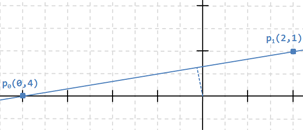Example: Calculate the standard form for p0 = (-4, 0) and p1 = (2, 1)
a = y0 - y1 = -1
b = x1 - x0 = 2 - (-4) = 6
c = x1*y0 - x0*y1 = 2 * 0 - (-4) * 1 = 4
So the standard form is -x + 6y = 4.
The visual representation is shown in the right figure. Normalizing the vector (a, b) results in (very approximated) roughly -0.16x + 0.98y = 0.66 which also matches with the figure.
Finally, it can also be converted to point-slope which results in y = 1/6x + 4/6.

Another, quite interesting way, is to retrieve the standard form from two points geometrically. As described above, the components a and b form the unit vector which is perpendicular to the line, i.e. (a,b) is the normal to the given line and c is the (perpendicular) distance from the line to the origin.
From the given points, the tangent of the line can be retrieved which is equal to the vector from p0 to p1, i.e. vector p1p0. This vector is normalized and rotated by 90 degrees, resulting in the normalized unit vector to the line. The components of this vector are the component a and b.
Finally, the value c is calculated by the dot product of this normal vector with one of the two given points. This works as a point can also be seen as vector from the origin to this point. And as described in , the dot product calculate the projection of one vector onto another vector. Because the vector p1p0 is already normalized, the result of the dot product is the length of the projected vector from origin to one of two points to the normal vector which is equal to the perpendicular distance from the origin to the line - in short words, the result is c.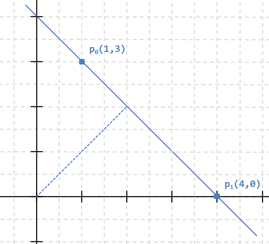Example: Get the standard form for p0 = (1, 3) and p1 = (4, 0)
The standard form, calculated the same way as above, is 3x + 3y = 12 which, divided by 3, is x + y = 4.
Consider now the geometric way:
1. The tangent vector of vector p1p0 is (4-1, 0-3) = (3, -3). The length is √ (32 + (-3)2) = √(18).
The normalize tangent vector is (3/√(18), -3/√(18)) that can be simplified to (3/√(9*2), -3/√(9*2)) = (1/√(2), -1/√(2)).

2. The rotation of this vector by 90 degrees can be generally be computed with a rotation matrix. In the same case of 2D, the rotation by 90 degrees is simply the exchange of the x and y value and the negation of one of them (see  for details):
This gives the unit normal vector (1/√(2), 1/√(2)), so a = 1/√(2) and b = 1/√(2).

3. Finally, to calculate c, the dot product of this vector with one of the two given points of the line is calculated.
By choosing p0 = (1, 3), this given c = 1 * 1/√(2) + 3 * 1/√(2) = 4/√(2). In total, we have 1/√(2) * x + 1/√(2) * y = 4/√(2). This represents the exactly same line as x + y + 4, just multiplied with √(2).
(Note that choosing p1 would result in the same value for c = 0 * 1/√(2) + 4 * 1/√(2) = 4/√(2).)

A final note: Rotating a vector by 90 degrees has two different results, depending on if the rotation is clockwise or counter-clockwise. Therefore, the other possible outcome of the rotated unit vector would be (-1/√(2), -1/√(2)) - this is the same vector, just negated, thus pointing into the opposite direction.
In this case, c would then be calculated as 1 * -1/√(2) + 3 * -1/√(2) = -4/√(2).
The retrieved standard form is then -1/√(2) * x - 1/√(2) * y = -4/√(2) - again the same line, the equation is just multiplied with -1.

# 6. General form

The general form of a line has following equation:

a * x + b * y + c = 0

Directly obvious is the close resemblance to the standard form. The general form is retrieved from a given standard form by moving the c component to the other side. For example, the line from above in standard form 3 * x + 4 * y = 6 is converted to general form by subtracting 6, resulting in 3 * x + 4 * y - 6 = 0.
Due to great similarity and easy transformation, the description of the previous chapter also applies to the general form.
The advantage of the general form is its ability to partition the space, meaning it easily provides the information for any point p = (x, y) if it lies directly on the line, on the right side of the line or on the left side.
First the easy case: If plugging p = (x, y) into the equation and the result is zero, then the line is on the line.
However, what means right or left of the line? Let's examine an example line for this.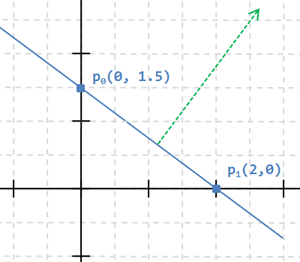Example:
The line right has general form 3 * x + 4 * y - 6 = 0.
The points p0 = (0, 1.5) lies on the line because 3 * 0 + 4 * 1.5 - 6 is zero as well as p1 = (2, 0) because 3 * 2 + 4 * 0 - 6 is zero. But also p = (-10, 9) because 3 * (-10) + 4 * 9 - 6 = 0.

But what about p = (1, 2) which definitely lies "above" the line?
Plugging it into the equation gives 3 * 1 + 4 * 2 - 6 = 5 which is positive.

Contrary, p = (-1, 0.5) is obviously "below" the line.
Plugging it into the line equation gives 3 * (-1) + 4 * 0.5 - 6 = -7 which is negative.

Note that the normal of this line is (3, 4) denoted in green in the figure on the right.

Summarized, the general form partitions the 2D space into two regions. Any point can be tested in which region it lies:

• If the result is 0, it lies directly on the line.
• If the result is > 0, then the point lies on the side where the normal of the line (given by the line equation) points to.
• If the result is < 0, then the point lies on the opposite side where the normal points to.

The reason of this useful behavior is established by the properties of the dot product (see also  for details).
The dot product of vectors v1 and v2 is v1x * v2x + v1y * v2y. It is zero if the two vectors are perpendicular. It is positive if the two vectors have an angle smaller than 90 degrees and negative if the angle is greater than 90 degrees.
Considering the general form a * x + b * y + c, it is quite similar to the dot product except for the component c. It has also been described above that (a, b) is actually the normal vector of the line. The point p = (x, y) to be tested can also be seen as a vector. So actually the dot product of the normal vector of the line and the vector to be tested is calculated, just with a fixed constant offset c.

# 7. Parametric form

The parametric form to define a line is a more geometrical approach using vectors. A vector defines a direction (and a magnitude) but it has no starting point. So a vector on its own can be used to specify the direction of a line, but no single specific line because there are an infinite number of lines having the same direction - they are all parallel. So one point of the line is additionally needed to define the line uniquely.

A given point on the line p0 can be treated as a vector p0 from the origin. The direction vector v describes the direction of the line.
So a vector to each point on the line, denoted as p, can be described by vector p0 to which a multiple of the v is added:
p = p0 + t * v
where t is a scalar value. Often v is a unit vector but this is not required.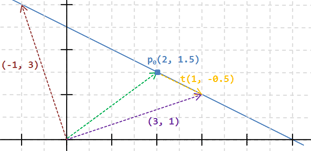Example:
In the figure on the right p0 is given as (2, 1.5) and t is (1, -0.5).
So a vector to each point on the line is given as p = (2, 1.5) + t * (1, -0.5).
The purple vector that points to a point on the line is (3, 1) that can be retrieved using t = 1:
(2, 1.5) + 1 * (1, -0.5) = (3, 1).
Similar, the dark red vector (-1, 3) is (2, 1.5) + -3 * (1, -0.5) with t = 3.

The x and y components can also be treated component-wise, thus:
p.x = p0.x + t * v.x
p.y = p0.y + t * v.y

So getting the parametric form from two points p0 and p1 is pretty easy.
Treating both points as vectors, the direction vector is just the difference, thus p1 - p0.
As reference point, just one of the two given points is used. Summarized:
p = p0 + t * (p1 - p0)Example:
Given two points p0 = (-2, 1) and p1 = (4, 2), the parametric form of the line is:
p = (-2, 1) + t * ((4, 2) - (-2, 1)) = (-2, 1) + t * (6, 1).
In components-wise , this is: p.x = -2 + 6 * t.
p.y = 1 + t.

Note that when setting t = 0, the result is p0. Using t = 1, the result is p1.
Another useful property is that the direction vector v directly provides the slope m = v.y / v.x. With the use of given point p0 it is straight-forward to convert it to the slope-intercept form. Also the transformation to any other form is easy by calculating p0 with t = 0 and p1 with t = 1 and use these two points to calculate any other line representation form.

## 8. Conclusion & References

This is my summary about the different ways of describing two-dimensional lines. Hope you enjoyed it and learned something!

Sunshine, February 2022

#### History

• 2022/04/14: Initial version.